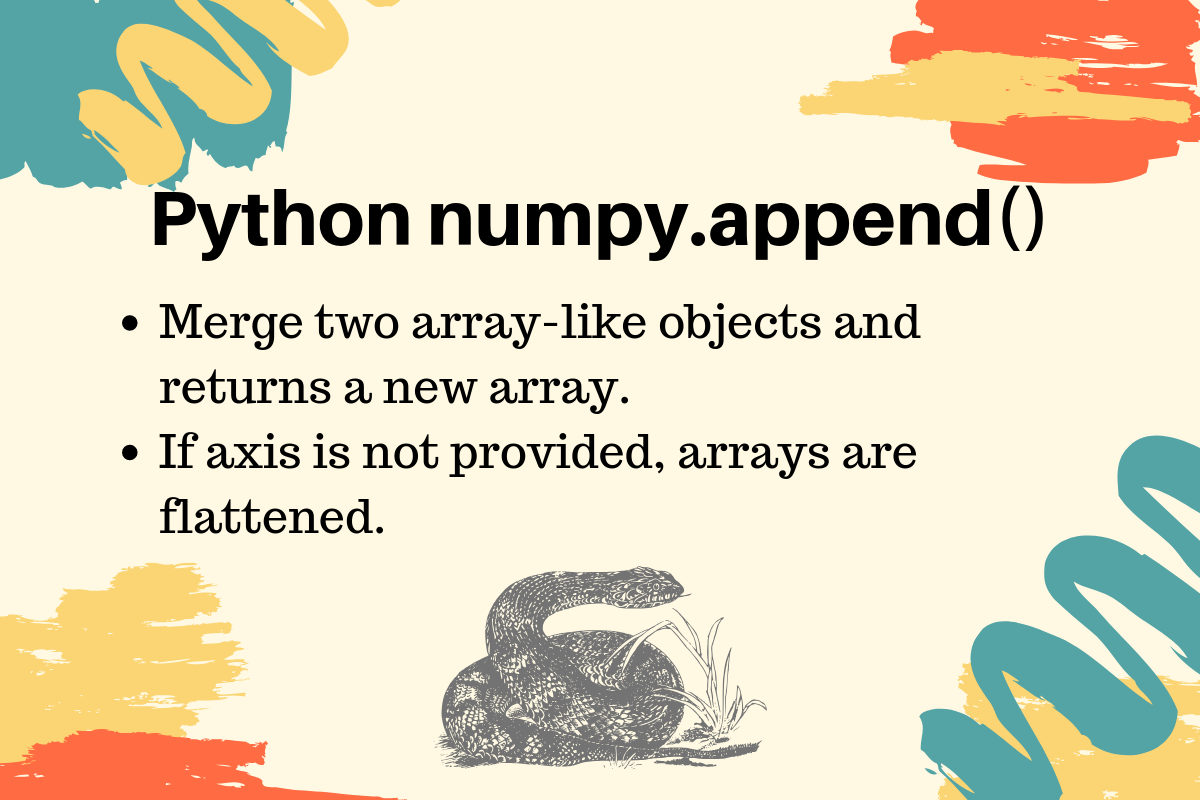# numpy.append() in Python

Published on August 3, 2022By PankajWhile we believe that this content benefits our community, we have not yet thoroughly reviewed it. If you have any suggestions for improvements, please let us know by clicking the “report an issue“ button at the bottom of the tutorial.

Python numpy append() function is used to merge two arrays. This function returns a new array and the original array remains unchanged.

## NumPy append() Syntax

The function syntax is:

``````numpy.append(arr, values, axis=None)
``````
• The arr can be an array-like object or a NumPy array. The values are appended to a copy of this array.
• The values are array-like objects and it’s appended to the end of the “arr” elements.
• The axis specifies the axis along which values are appended. If the axis is not provided, both the arrays are flattened.

## Python numpy.append() Examples

Let’s look at some examples of NumPy append() function.

### 1. Flattening Two Arrays

``````import numpy as np

arr1 = np.array([[1, 2], [3, 4]])
arr2 = np.array([[10, 20], [30, 40]])

# no axis provided, array elements will be flattened
arr_flat = np.append(arr1, arr2)

print(arr_flat)  # [ 1  2  3  4 10 20 30 40]
``````

### 2. Merging Along Axis

``````import numpy as np

arr_merged = np.append([[1, 2], [3, 4]], [[10, 20], [30, 40]], axis=0)
print(f'Merged 2x2 Arrays along Axis-0:\n{arr_merged}')

arr_merged = np.append([[1, 2], [3, 4]], [[10, 20], [30, 40]], axis=1)
print(f'Merged 2x2 Arrays along Axis-1:\n{arr_merged}')
``````

Output:

``````Merged 2x2 Arrays along Axis-0:
[[ 1  2]
[ 3  4]
[10 20]
[30 40]]
Merged 2x2 Arrays along Axis-1:
[[ 1  2 10 20]
[ 3  4 30 40]]
``````
• When the 2x2 arrays are merged along the x-axis, the output array size is 4x2.
• When the 2x2 arrays are merged along the y-axis, the output array size is 2x4.

### 3. Merging Arrays of different shapes

The append() function throws ValueError if both the arrays are of different shape, excluding the axis. Let’s understand this scenario with a simple example.

``````arr3 = np.append([[1, 2]], [[1, 2, 3], [1, 2, 3]])
print(arr3)

arr3 = np.append([[1, 2]], [[1, 2], [3, 4]], axis=0)
print(arr3)
``````
• In the first example, the array elements are flattened. So even if they have completely different size - 1x2 and 2x3, the append() works fine.
• In the second example, the array shapes are 1x2 and 2x2. Since we are appending along the 0-axis, the 0-axis shape can be different. The other shapes should be the same, so this append() will also work fine.

Output:

``````[1 2 1 2 3 1 2 3]

[[1 2]
[1 2]
[3 4]]
``````

Let’s look at another example where ValueError will be raised.

``````>>> import numpy as np
>>>
>>> arr3 = np.append([[1, 2]], [[1, 2, 3]], axis=0)
Traceback (most recent call last):
File "", line 1, in
File "/Library/Frameworks/Python.framework/Versions/3.7/lib/python3.7/site-packages/numpy/lib/function_base.py", line 4528, in append
return concatenate((arr, values), axis=axis)
ValueError: all the input array dimensions except for the concatenation axis must match exactly
>>>
``````

The array shapes are 1x2 and 2x3. Since the axis-1 shapes are different, ValueError is raised. Reference: API Doc

Thanks for learning with the DigitalOcean Community. Check out our offerings for compute, storage, networking, and managed databases.Pankaj

author

#### Still looking for an answer?

Ask a questionSearch for more help

JournalDev
DigitalOcean EmployeeJuly 31, 2020

Nice tutorial man! people don’t understand this basic stuff sometimes but with simple examples and little but still useful explanations like yours, everyone can get it!

- Andoni

JournalDev
DigitalOcean EmployeeFebruary 10, 2020

I have now learned to numpy append

- numpyappend

Click below to sign up and get \$200 of credit to try our products over 60 days!

### Popular Topics##### Hollie's Hub for Good

Working on improving health and education, reducing inequality, and spurring economic growth? We’d like to help.##### Become a contributor

You get paid; we donate to tech nonprofits.

##### Welcome to the developer cloud

DigitalOcean makes it simple to launch in the cloud and scale up as you grow – whether you’re running one virtual machine or ten thousand.© 2023 DigitalOcean, LLC.